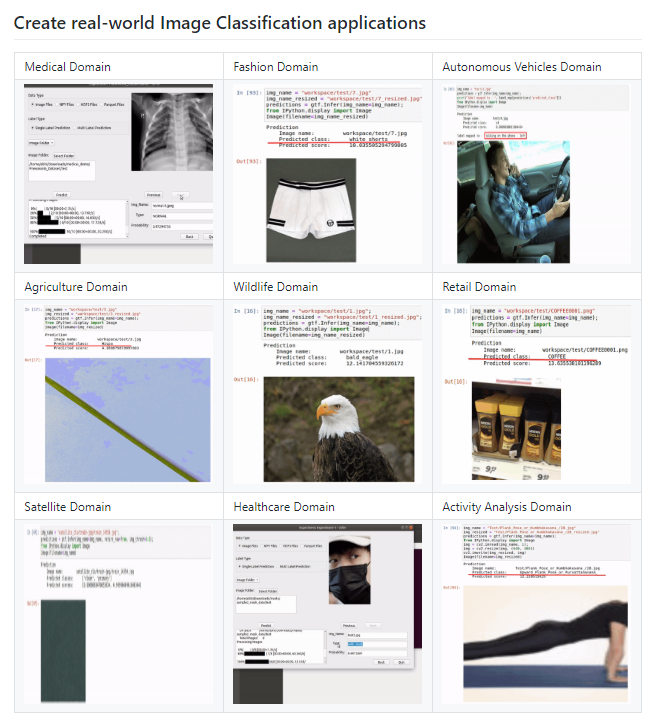# Image Classifier — Zalando Clothing Store using Monk Library`ktf.Train()`

## a. Analyse Learning Rates

`# Analysis Project Nameanalysis_name = "analyse_learning_rates_vgg16"# Learning rates to explorelrs = [0.1, 0.05, 0.01, 0.005, 0.0001]# Number of epochs for each sub-experiment to runepochs=5# Percentage of original dataset to take in for experimentation# We're taking 5% of our original dataset.percent_data=5# I made sure that all the GPU processors are runningktf.update_num_processors(2)# Very important to reload post updatingktf.Reload()`

Output:

`# "keep_all" - Keep all the sub experiments created# "keep_none" - Delete all sub experiments createdanalysis = ktf.Analyse_Learning_Rates(analysis_name, lrs, percent_data, num_epochs=epochs, state="keep_none")`

Output:

## Result

From the above table, it is clear that `Learning_Rate_0.0001` has the least validation loss. We will update our learning rate with this.

`ktf.update_learning_rate(0.0001)# Very important to reload post updates ktf.Reload()`

## b. Analyse Batch sizes

`# Analysis Project Nameanalysis_name = "analyse_batch_sizes_vgg16"# Batch sizes to explorebatch_sizes = [2, 4, 8, 12]# Note: We're using the same percent_data and num_epochs.# "keep_all" - Keep all the sub experiments created# "keep_none" - Delete all sub experiments createdanalysis_batches = ktf.Analyse_Batch_Sizes(analysis_name, batch_sizes, percent_data, num_epochs=epochs, state="keep_none")`

## Result

From the above table, it is clear that `Batch_Size_12` has the least validation loss. We will update the model with this.

`ktf.update_batch_size(12)# Very important to reload post updatesktf.Reload()`

## c. Analyse Optimizers

`# Analysis Project Nameanalysis_name = "analyse_optimizers_vgg16"# Optimizers to exploreoptimizers = ["sgd", "adam", "adagrad"]# "keep_all" - Keep all the sub experiments created# "keep_non" - Delete all sub experiments createdanalysis_optimizers = ktf.Analyse_Optimizers(analysis_name, optimizers, percent_data, num_epochs=epochs, state="keep_none")`

## Result

From the above table, it is clear that we should go for `Optimizer_adagrad` since it has the least validation loss.

## Summary of Hyperparameter Tuning Experiment

Here ends our experiment and now it’s time to switch on the Expert Mode to train our Classifier using the above Hyperparameters.

Summary:

• Learning Rate — 0.0001
• Batch size — 12

# 1. Training from scratch: vgg16

## Expert Mode

Let’s create another Experiment named expert_mode_vgg16 and train our classifier from scratch.

`ktf = prototype(verbose=1)ktf.Prototype("Project-Zalando", "expert_mode_vgg16")ktf.Dataset_Params(dataset_path="/content/drive/My Drive/Data/zalando", split=0.8, input_size=224, batch_size=12, shuffle_data=True, num_processors=2)# Load the datasetktf.Dataset()ktf.Model_Params(model_name="vgg16", freeze_base_network=True, use_gpu=True, use_pretrained=True)ktf.Model()ktf.Training_Params(num_epochs=5, display_progress=True, display_progress_realtime=True, save_intermediate_models=True, intermediate_model_prefix="intermediate_model_", save_training_logs=True)# Update optimizer and learning ratektf.optimizer_adagrad(0.0001)ktf.loss_crossentropy()# Trainingktf.Train()`

Output:

## Validation

`ktf = prototype(verbose=1);ktf.Prototype("Project-Zalando", "expert_mode_vgg16", eval_infer=True)# Just for example purposes, validating on the training set itselfktf.Dataset_Params(dataset_path="/content/drive/My Drive/Data/zalando")ktf.Dataset()accuracy, class_based_accuracy = ktf.Evaluate()`

## Inference

Let’s see the Prediction on sample images.

`ktf = prototype(verbose=1)ktf.Prototype("Project-Zalando", "expert_mode_vgg16", eval_infer=True)`

`img_name = "/content/1FI21J00A-A11@10.jpg"predictions = ktf.Infer(img_name=img_name)#Display from IPython.display import ImageImage(filename=img_name)`